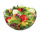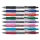# Fraction calculator

The calculator performs basic and advanced operations with fractions, expressions with fractions combined with integers, decimals, and mixed numbers. It also shows detailed step-by-step information about the fraction calculation procedure. Solve problems with two, three, or more fractions and numbers in one expression.

## Result:

### 17/3 = 17/3 = 5 2/3 ≅ 5.6666667

Spelled result in words is seventeen thirds (or five and two thirds).

#### Rules for expressions with fractions:

Fractions - use the slash “/” between the numerator and denominator, i.e., for five-hundredths, enter 5/100. If you are using mixed numbers, be sure to leave a single space between the whole and fraction part.
The slash separates the numerator (number above a fraction line) and denominator (number below).

Mixed numerals (mixed fractions or mixed numbers) write as non-zero integer separated by one space and fraction i.e., 1 2/3 (having the same sign). An example of a negative mixed fraction: -5 1/2.
Because slash is both signs for fraction line and division, we recommended use colon (:) as the operator of division fractions i.e., 1/2 : 3.

Decimals (decimal numbers) enter with a decimal point . and they are automatically converted to fractions - i.e. 1.45.

The colon : and slash / is the symbol of division. Can be used to divide mixed numbers 1 2/3 : 4 3/8 or can be used for write complex fractions i.e. 1/2 : 1/3.
An asterisk * or × is the symbol for multiplication.
Plus + is addition, minus sign - is subtraction and ()[] is mathematical parentheses.
The exponentiation/power symbol is ^ - for example: (7/8-4/5)^2 = (7/8-4/5)2

#### Examples:

adding fractions: 2/4 + 3/4
subtracting fractions: 2/3 - 1/2
multiplying fractions: 7/8 * 3/9
dividing Fractions: 1/2 : 3/4
exponentiation of fraction: 3/5^3
fractional exponents: 16 ^ 1/2
adding fractions and mixed numbers: 8/5 + 6 2/7
dividing integer and fraction: 5 ÷ 1/2
complex fractions: 5/8 : 2 2/3
decimal to fraction: 0.625
Fraction to Decimal: 1/4
Fraction to Percent: 1/8 %
comparing fractions: 1/4 2/3
multiplying a fraction by a whole number: 6 * 3/4
square root of a fraction: sqrt(1/16)
reducing or simplifying the fraction (simplification) - dividing the numerator and denominator of a fraction by the same non-zero number - equivalent fraction: 4/22
expression with brackets: 1/3 * (1/2 - 3 3/8)
compound fraction: 3/4 of 5/7
fractions multiple: 2/3 of 3/5
divide to find the quotient: 3/5 ÷ 2/3

The calculator follows well-known rules for order of operations. The most common mnemonics for remembering this order of operations are:
PEMDAS - Parentheses, Exponents, Multiplication, Division, Addition, Subtraction.
BEDMAS - Brackets, Exponents, Division, Multiplication, Addition, Subtraction
BODMAS - Brackets, Of or Order, Division, Multiplication, Addition, Subtraction.
GEMDAS - Grouping Symbols - brackets (){}, Exponents, Multiplication, Division, Addition, Subtraction.
Be careful, always do multiplication and division before addition and subtraction. Some operators (+ and -) and (* and /) has the same priority and then must evaluate from left to right.

## Fractions in word problems:

• A quotientWhat is the quotient of 3/10 divided by 2/4 as a fraction?
• Berry SmoothieRory has 5/8 cup of milk. How much milk does she have left after she doubles the recipe of the smoothie? Berry Smoothie: 2 cups strawberries 1 cup blueberries 1/4 cup milk 1 tbsp (tablespoon) sugar 1/2 tsp (teaspoon) lemon juice 1/8 tsp (teaspoon) vanilla
• Equation with xSolve the following equation: 2x- (8x + 1) - (x + 2) / 5 = 9
• Fraction of a fractionWhat is two-quarters of twelve?
• RegroupingSubtract mixed number with regrouping: 11 17/20- 6 19/20
• Unknown numberFind the unknown number equal to a quarter of a fifth of a number, which is by 152 more than an unknown number.
• Adding mixed 3Why does 1 3/4 + 2 9/10 equal 4.65? How do you solve this?
• Two pizzasJacobs mom bought two whole pizzas. He ate 2/10 of the pizza and his dad ate 1 1/5. How much is left.
• Mrs. SusanMrs. Susan bought 1/8 m of curtain cloth. She used 3/5 m to make a curtain for the living room window. How many meters of cloth were not used?
• MidnightHow many hours are, if the time that elapsed since 8:00 is 2/5 of the time that will past till midnight?
• SaladWe need two tenths kg of a carrot, one tenth of peas and three tenths of of tomatoes to make salad. Express the fraction of the weight of the vegetables to be salad. Convert the result to grams.
• Giraffes to monkeysThe ratio of the number of giraffes to the number of monkeys in a zoo is 2 to 5. Which statement about the giraffes and monkeys could be true? A. For every 10 monkeys in the zoo, there are 4 giraffes. B. For every  giraffe in the zoo, there are 3 monkeys.
• Two penTwo pens and one notebook costs \$56. One pen costs 1/5 of one notebook. How much is the one notebook?